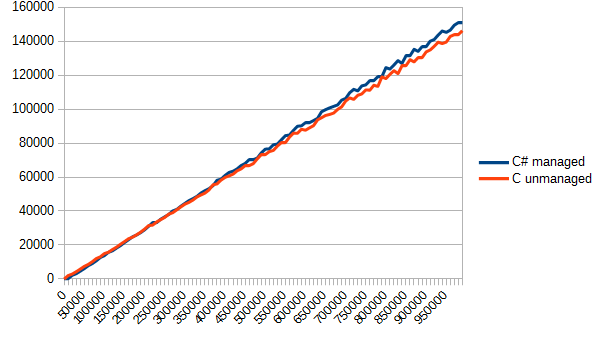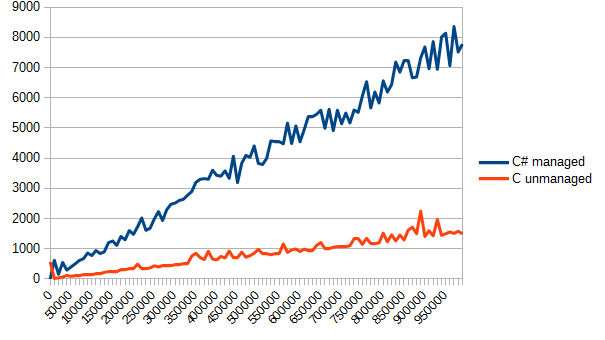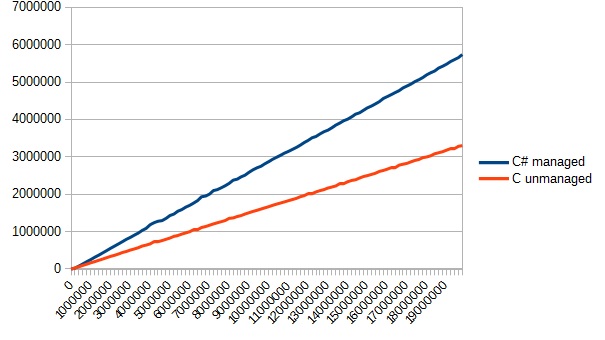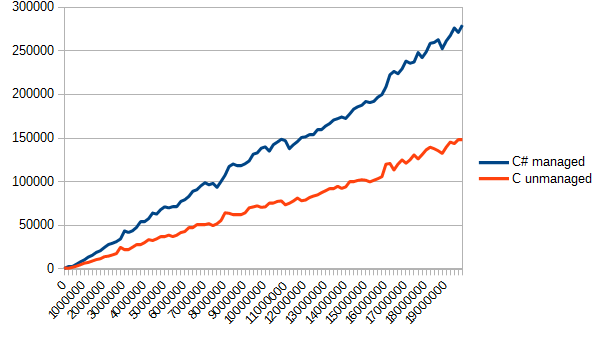# .NET Managed + C Unmanaged: What’s the Cost?

Total: 1 Average: 5

When I was programming in C#, I used to send all recursive tasks to an unmanaged C code, since the .NET performance was problematic. And now, looking back at my past experience, I think of the benefits of such code division. Do I really benefit from it, and if yes, how much? What is the best way of building API with such approach?

## Why?

Developing a project in two different languages is a very dubious thing. Moreover, the unmanaged code is really difficult in terms of implementation, debugging and maintenance. But a chance to implement the functionality that can work faster is already worth considering, especially when it comes to critical and important areas or high-load applications.

Another possible answer: functionality has been implemented in the unmanaged mode. Why should I rewrite the entire solution, if I can quickly wrap everything into .NET and use it from there?

Lyrics

The code was written in Visual Studio Community 2015. For evaluation, I used my PC with i5-3470, the 12 Gb dual channel 1333MHz RAM, and the 7200 rpm hard disc. Measuring was carried out with the help of System.Diagnostics.Stopwatch that is more precise than DateTime, since it is implemented over PerformanceCounter. Tests were run on the Release variants of assemblies to make the results maximally real. I used .NET 4.5.2, and the C++ project was compiled with the /TC (Compile as C) option enabled.

## Calling Functions

I began my research from the evaluation of the function call speed. There are several reasons for this. Firstly, we need to call functions anyways, and functions are called from the loaded dll slowly in comparison with the code in the same module. Secondly, most of existing C#-wrappings over any unmanaged code are implemented in a similar way (for instance, sharpgl, openal-cs). In fact, it is the most obvious and the simplest way to embed the unmanaged code.

Before evaluation, we need to think how to store and evaluate the measurement results. I selected «CSV!» and wrote a simple class for storing data in this format:

```    public class CSVReport : IDisposable
{
int columnsCount;
StreamWriter writer;
public CSVReport(string path, params string[] header)
{
writer = new StreamWriter(path);
for (int i = 1; i < header.Length; i++)
writer.Write("\r\n");
}

public void Write(params object[] values)
{
if (values.Length != columnsCount)
throw new ArgumentException("Columns count for row didn't match table columns count");
writer.Write(values.ToString());
for (int i = 1; i < values.Length; i++)
writer.Write("," + values[i].ToString());
writer.Write("\r\n");
}

public void Dispose()
{
writer.Close();
}
}```

It can hardly be considered as a functional variant, but it perfectly suits my needs. For testing, I wrote a simple class that can only sum numbers and store the results. Here is how it looks like:

```    class Summer
{
public int Sum
{
get; private set;
}

public Summer()
{
Sum = 0;
}

{
Sum += a;
}

public void Reset()
{
Sum = 0;
}
}```

But it is a managed variant, and we need an unmanaged one as well. So, I created a template dll project and added a file to it, for instance, api.h with the export definition:

```#ifndef _API_H_
#define _API_H_

#define EXPORT  __declspec(dllexport)
#define STD_API __stdcall

#endif```

Let’s put the summer.c class next to it and implement all functionality we need:

```#include "api.h"

int sum;

EXPORT void STD_API summer_init( void )
{
sum = 0;
}

EXPORT void STD_API summer_add( int value )
{
sum += value;
}

EXPORT int STD_API summer_sum( void )
{
return sum;
}```

Now, we need a wrapper class over this mess:

```    class SummerUnmanaged
{
const string dllName = @"unmanaged_test.dll";
[DllImport(dllName)]
private static extern void summer_init();

[DllImport(dllName)]
private static extern void summer_add(int v);

[DllImport(dllName)]
private static extern int summer_sum();

public int Sum
{
get
{
return summer_sum();
}
}

public SummerUnmanaged()
{
summer_init();
}

{
}

public void Reset()
{
summer_init();
}
}```

As a result, we got exactly what we wanted. There are two implementations that are similar in terms of usage: one is in C#, and the second one is in C. Now, we can look at them, and check the results! Let’s write a code for measuring execution time for n calls of the same class:

```        static void TestCall()
{
Console.WriteLine("Function calls...");
Stopwatch sw = new Stopwatch();
Summer s_managed = new Summer();
SummerUnmanaged s_unmanaged = new SummerUnmanaged();
Random r = new Random();
int[] data;
CSVReport report = new CSVReport("fun_call.csv", "elements", "C# managed", "C unmanaged");
data = new int;
for (int j = 0; j < 1000000; j++)
data[j] = r.Next(-1, 2); // Generating trash

for (int i=0; i<100; i++)
{
// To be independent of the console
Console.Write("\r{0}/100", i+1);
int length = 10000*i;
long managedTime = 0, unmanagedTime = 0;
s_managed.Reset();
sw.Start();
for (int j = 0; j < length; j++)
{
}
sw.Stop();
managedTime = sw.ElapsedTicks;
sw.Reset();
sw.Start();
for(int j=0; j<length; j++)
{
}
sw.Stop();
unmanagedTime = sw.ElapsedTicks;
report.Write(length, managedTime, unmanagedTime);
}
report.Dispose();
Console.WriteLine();
}```

The only thing left is to call this function somewhere from main and take a look at fun_call.csv. I don’t want to bother you with numbers, so here is a chart with time in ticks (in the vertical direction) and the number of the function calls (in the horizontal direction).The result surprised me a bit. C# was a leader of this test. However, in spite of the same moduleand the possibility to inline, both variants turned out to be quite similar. In this particular case, code division turned out to be useless – we didn’t benefit from it at all, and complicated the project.

## Arrays

After a short analysis of the results, I understood that I need to send data not by one element, but in arrays. So, it’s time to update the code. Let’s add the functionality:

```        public void AddMany(int[] data)
{
int length = data.Length;
for (int i = 0; i < length; i++)
Sum += i;
}```

And here is the C part:

```EXPORT int STD_API summer_add_many( int* data, int length )
{
for ( int i = 0; i < length; i++ )
sum += data[ i ];
}

[DllImport(dllName)]
private static extern void summer_add_many(int[] data, int length);

{
}```

So, I had to rewrite the performance evaluation function. The full version is provided below. In few words, now we generate an array of n random elements and call the function to add them.

```        static void TestArrays()
{
Console.WriteLine("Arrays...");
Stopwatch sw = new Stopwatch();
Summer s_managed = new Summer();
SummerUnmanaged s_unmanaged = new SummerUnmanaged();
Random r = new Random();
int[] data;
CSVReport report = new CSVReport("arrays.csv", "elements", "C# managed", "C unmanaged");

for (int i = 0; i < 100; i++)
{
Console.Write("\r{0}/100", i+1);
int length = 10000 * i;
long managedTime = 0, unmanagedTime = 0;
data = new int[length];
for (int j = 0; j < length; j++) // generate hash
data[j] = r.Next(-1, 2);
s_managed.Reset();
sw.Start();
sw.Stop();
managedTime = sw.ElapsedTicks;
sw.Reset();
sw.Start();
sw.Stop();
unmanagedTime = sw.ElapsedTicks;
report.Write(length, managedTime, unmanagedTime);
}
report.Dispose();
Console.WriteLine();
}```

Now, let’s run it and check the report. Time in ticks is in the vertical direction, and the number of the array elements is in the horizontal direction.Obviously, C copes with a simple array processing much better. But it is the cost of manageability. While managed code will throw an exception in the case of overflow or violation of array bounds, C can simply rewrite not its own memory.

When it became clear that C could process big arrays a way faster, I decided that files must be read. I wanted to check the speed of code interaction with the system.

For this, I generated a stack of files (which size grows linearly).

```        static void Generate()
{
Random r = new Random();
for(int i=0; i<100; i++)
{
BinaryWriter writer = new BinaryWriter(File.OpenWrite("file" + i.ToString()));
for(int j=0; j<200000*i; j++)
{
writer.Write(r.Next(-1, 2));
}
writer.Close();
Console.WriteLine("Generating {0}", i);
}
}```

As a result, the largest file had the size of 75 Mb, which is quite ok. For testing, I didn’t create a separate class and wrote code right in the main class.

```        static int FileSum(string path)
{
int sum = 0;
long length = br.BaseStream.Length;
while(br.BaseStream.Position != length)
{
}
br.Close();

return sum;
}```

As you can see from the code, the task was to sum up all integers from the file.

Here is the corresponding implementation in C:

```EXPORT int STD_API file_sum( const char* path )
{
FILE *f = fopen( path, "rb" );
if ( !f )
return 0;
int sum = 0;
while ( !feof( f ) )
{
}
fclose( f );
return sum;
}```

Now, we only need to read all files cyclically and change the work speed for each implementation. Here is a chart with the results.As you can see from the chart, C turned out to be much faster (approximately, by half).

### Return of Arrays

The next step of measuring performance was the return of more complex types, since usage of integers and floating point numbers is not always convenient. So, we need to check the speed of transformation of unmanaged memory areas to managed ones. For this, I decided to implement a simple task: read of the entire file and return of its content as a byte array.

On a clean C#, such task can be implemented in a pretty simple way, but linking of the C code with the C# code in this case required extra thinking.

Firstly, a solution in C#:

```        static byte[] FileRead(string path)
{
br.Close();
return ret;
}```

And the corresponding solution in C:

```EXPORT char* STD_API file_read( const char* path, int* read )
{
FILE *f = fopen( path, "rb" );
if ( !f )
return 0;

fseek( f, 0, SEEK_END );
long length = ftell( f );
fseek( f, 0, SEEK_SET );

int sum = 0;
uint8_t *buffer = malloc( length );
fclose( f );

return buffer;
}```

For a successful call of such function from C#, we need to write a wrapper that calls this function, copies data from the unmanaged memory to the managed one and frees the unmanaged area:

```        static byte[] FileReadUnmanaged(string path)
{
int length = 0;
IntPtr unmanaged = file_read(path, ref length);
byte[] managed = new byte[length];
Marshal.Copy(unmanaged, managed, 0, length);
Marshal.FreeHGlobal(unmanaged);
return managed;
}```

As for the measurement functions, only the corresponding calls of the measured functions were changed. The result looks as follows:Even with the time spent on memory copying, C again became a leader and executed the task twice faster. Frankly speaking, I expected a bit different results (taking into account the second test). The reason is that data read in C# takes much time. As for C, time is spent on copying from the unmanaged memory to the managed one.

A logical conclusion of all tests I performed was to implement the full-featured algorithm in C# and C and to evaluate performance.

As an algorithm, I used the read of the uncompressed TGA file with 32 bits per pixel and its transformation into the RGBA view (the TGA format supposes storing of color as BGRA). In addition, we will return not bytes, but the Color structures.

```        struct Color
{
public byte r, g, b, a;
}```

Implementation of the algorithm is quite heavy and is hardly interesting.

```        static Color[] TGARead(string path)
{
int width = (header << 8) + header;  // A small lifehack to get short
int height = (header << 8) + header; // Little-Endian, less significant digint goes first
byte[] data;
data = br.ReadBytes(width * height * 4);
Color[] colors = new Color[width * height];
for(int i=0; i<width*height*4; i+=4)
{
int index = i / 4;
colors[index].b = data[i];
colors[index].g = data[i + 1];
colors[index].r = data[i + 2];
colors[index].a = data[i + 3];
}
br.Close();
return colors;
}
{
int width = 0, height = 0;
IntPtr colors = tga_read(path, ref width, ref height);
IntPtr save = colors;
Color[] ret = new Color[width * height];
for(int i=0; i<width*height; i++)
{
ret[i] = Marshal.PtrToStructure<Color>(colors);
colors += 4;
}
Marshal.FreeHGlobal(save);
return ret;
}```

And here is a C variant:

```#include "api.h"

#include <stdlib.h>
#include <stdio.h>

// Causes problems, if the structure is not aligned
// by 4 bytes
typedef struct {
char r, g, b, a;
} COLOR;

// A hack to read the whole structure
#pragma pack(push)
#pragma pack(1)
typedef struct {
char idlength;
char colourmaptype;
char datatypecode;
short colourmaporigin;
short colourmaplength;
char colourmapdepth;
short x_origin;
short y_origin;
short width;
short height;
char bitsperpixel;
char imagedescriptor;
#pragma pack(pop)

EXPORT COLOR* tga_read( const char* path, int* width, int* height )
{
FILE *f = fopen( path, "rb" );
COLOR *colors = malloc( sizeof( COLOR ) * header.height * header.width );
for ( int i = 0; i < header.width * header.height; i++ )
{
char t = colors[ i ].r;
colors[ i ].r = colors[ i ].b;
colors[ i ].b = t;
}
fclose( f );

return colors;
}```

Now, we need to draw a simple TGA image and load it n times. The result is the following (time in ticks is in the vertical direction, and the number of file reads is in the horizontal direction):Note that I intentionally used the features of C in its favor. The read from file right to the structure has made my life much easier (in the cases, when structures are not aligned by 4 bytes, debugging will be really painful). However, I’m happy with the result. I managed to successfully implement a simple algorithm on C and then use it in C# with success. Thus, I got an answer to my initial question, we can really win, but not always. Sometimes, we can win a little, sometimes we cannot win at all, and sometimes, we can win a lot.

### Conclusion

The idea to pass implementation of something to another language is questionable, as I wrote at the very beginning. After all, you can hardly find many ways to implement this speed-up method. If the file opening freezes UI, you can send loading to a separate background tread, and then even a 1-second loading will not cause any troubles.

Correspondingly, it is worth the cost only when performance is really in need, and you cannot improve in any other way. Or, if there is a ready-to-use algorithm.

Note, that a simple wrapping over the unmanaged dll will not improve performance greatly, and all speed of the unmanaged languages can be revealed only during processing of large volumes of data.

C# perfectly copes with passing manageable resources to the unmanaged code, but the reverse change takes too much time. That is why it is better to avoid frequent data conversion and keep unmanageable resources in the unmanaged code. If there is no need in data modification/read in the managed code, you can use IntPtr for storing pointers and pass the rest to the unmanaged code.

Of course, we can (and should) make additional research before taking the final decision on passing code to the unmanaged assemblies. But the current information allows deciding, whether such actions are viable or not.

That’s it. Thank you for reading.

The article was translated by the CodingSight team with the permission of the author.